## Sonitypes Page

The following graphs are sonitypes (archetypes of basic sound representations of data) of different graphs. The graph's X axis is always represented by time in these sonified graphs. The Y axis of the data point is represented by the pitch of the piano instrument. The first and second derivatives of the data are represented with the drum beat. The frequency of the beat represents the slope (so the greater the slope, the more frequent the beat.) The second derivative is represented by the pitch of the drum beat, a low pitch indicates positive curvature (concave) and a high pitch indicates negative curvature (convex); Linear data is indicted with a medium pitch.

The data set from which the visual and auditory graphs were drawn, can be accessed by clicking on the visual graph.

Linear:
Graph of linearly increasing data: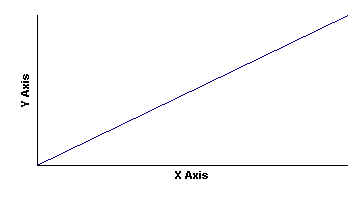```      Dowload
```

Graph of linearly decreasing data:```     Download
```

Curved:
Graph of log(x):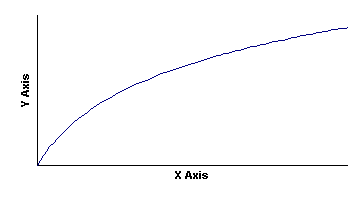```      Download
```

Graph of square root of x: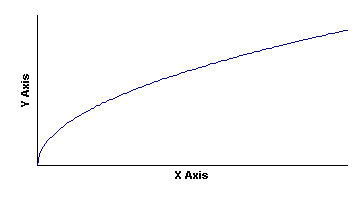```      Download
```

Graph of 1/x data: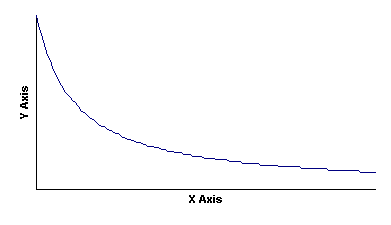```      Download
```

Graph of x squared: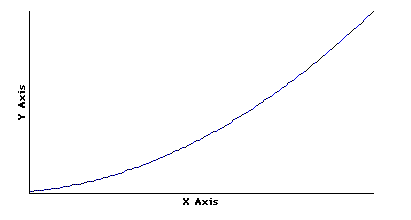```      Download
```

Graph of exponential data: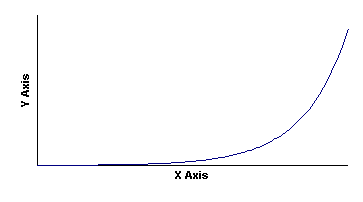```      Download
```

Graph of a Gaussian curve: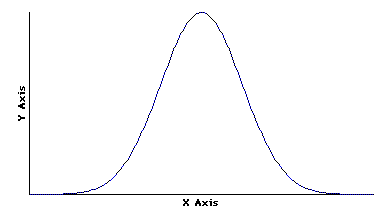```      Download
```

Negative data values (or Y axis values below a set-point) can be represented by changing the voice of the instrument; say from a piano to harpsichord or other tonal quality.

Here is an example of an audio graph of the function Sin(x). In this graph, the data, first derivative, and negative auditory indicators can be heard.